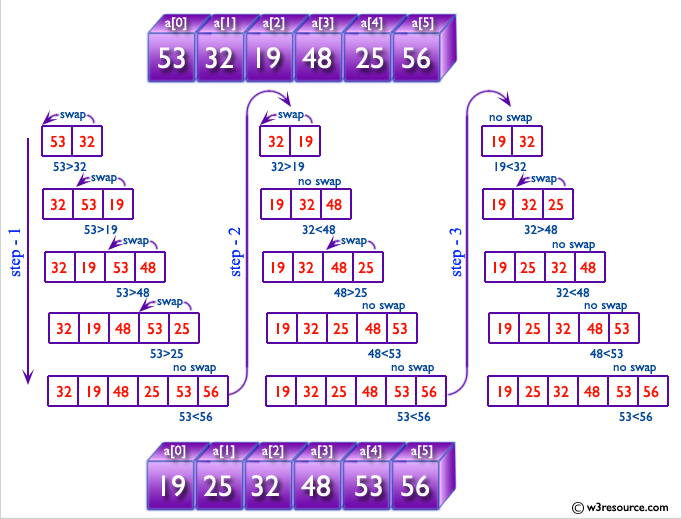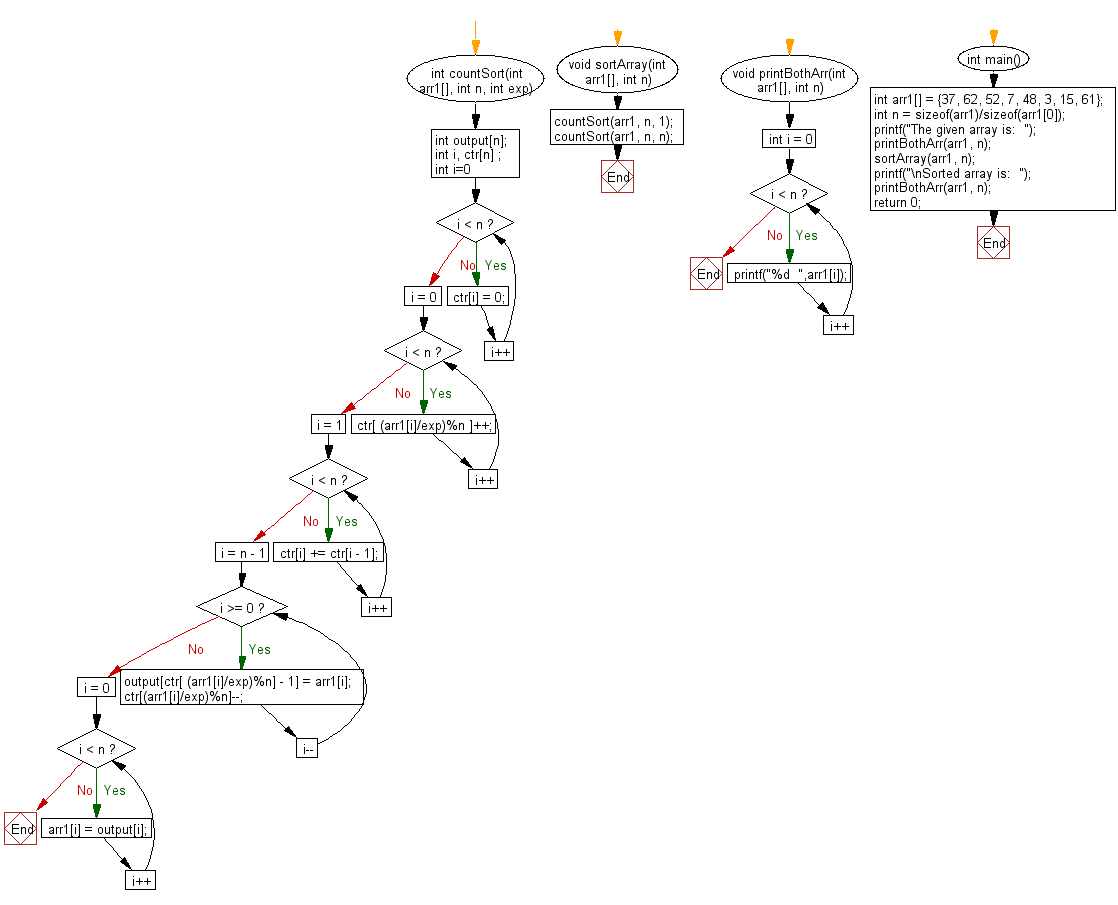﻿ C exercises: Sort n numbers in range from 0 to n^2 - w3resource# C Exercises: Sort n numbers in range from 0 to n^2

## C Array: Exercise-79 with Solution

Write a program in C to sort n numbers in range from 0 to n^2.

Pictorial Presentation:Sample Solution:

C Code:

``````#include <stdio.h>
int countSort(int arr1[], int n, int exp)
{
int output[n];
int i, ctr[n] ;
for (int i=0; i < n; i++)
ctr[i] = 0;

for (i = 0; i < n; i++)
ctr[ (arr1[i]/exp)%n ]++;

for (i = 1; i < n; i++)
ctr[i] += ctr[i - 1];

for (i = n - 1; i >= 0; i--)
{
output[ctr[ (arr1[i]/exp)%n] - 1] = arr1[i];
ctr[(arr1[i]/exp)%n]--;
}
for (i = 0; i < n; i++)
arr1[i] = output[i];
}

void sortArray(int arr1[], int n)
{
countSort(arr1, n, 1);
countSort(arr1, n, n);
}

void printBothArr(int arr1[], int n)
{
for (int i = 0; i < n; i++)
printf("%d  ",arr1[i]);
}
int main()
{
int arr1[] = {37, 62, 52, 7, 48, 3, 15, 61};
int n = sizeof(arr1)/sizeof(arr1);
printf("The given array is:  ");
printBothArr(arr1, n);
sortArray(arr1, n);
printf("\nSorted array is:  ");
printBothArr(arr1, n);
return 0;
}
```
```

Sample Output:

```The given array is:  37  62  52  7  48  3  15  61
Sorted array is:  3  7  15  37  48  52  61  62
```

Flowchart:C Programming Code Editor:

Improve this sample solution and post your code through Disqus.

What is the difficulty level of this exercise?

﻿